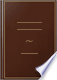Αναζήτηση Εικόνες Χάρτες Play YouTube Ειδήσεις Gmail Drive Περισσότερα »
Είσοδος
 Βιβλία Βιβλία 1 - 10 από 46 για The side of a regular hexagon inscribed in a circle is equal to the radius of the....The side of a regular hexagon inscribed in a circle is equal to the radius of the circle.Elements of Plane Geometry: For the Use of Schools - Σελίδα 84
των Nicholas Tillinghast - 1844 - 96 σελίδες
Πλήρης προβολή - Σχετικά με αυτό το βιβλίο## A New and Enlarged Military Dictionary: Or, Alphabetical Explanation of ...

Charles James - 1805 - 1006 σελίδες
...being fortified with б bastions. If the sides and angles be equal, it is called a regular hexagon. The side of a regular hexagon inscribed in a circle is equal to the radius of that circle; hum ea regular hexagon is inscribed in a circle, by setting the radius oí tí times upon the pelip'.iory:...## A Military Dictionary: Or, Explaination of the Several Systems of Discipline ...

William Duane - 1810 - 748 σελίδες
...being fortified with ft bastions. If the sides and angles be equal, it is called a regular hexagon. The side of a regular hexagon inscribed in a circle, is equal to the radius of that circle; hence a regular hexagon is inscribed in a circle, by setting the radius of 6 times upon the periphery:...## Euclid's Elements of Geometry: The Six First Books. To which are Added ...

Rev. John Allen - 1822 - 494 σελίδες
...equal (Cor. 2. 29. 3), which hexagon is therefore regular (Schol. 6. 4. and Def. 7. 4). Cor. 1. — The side of a regular hexagon, inscribed in a circle, is equal to the radius. For, in the above construction, AB one of the sides of the inscribed hexagon, is equal to the radius...## Euclid's Elements of Geometry: The Six First Books. To which are Added ...

Rev. John Allen - 1822 - 494 σελίδες
...(Cor. 2. 29. 3), which hexagon is therefore regular (Schol. 6. 4. and Def. 7. 4). It A. Cor. 1.—The side of a regular hexagon, inscribed in a circle, is equal to the radius. For, in the above construction, AB one of the sides of the inscribed hexagon, is equal to the radius...## Elements of Geometry: With Notes

John Radford Young - 1827 - 208 σελίδες
...equivalent in surface, circumscribed about equal circles, are also equal in perimeter. i PROPOSITION VI. THEOREM. The side of a regular hexagon inscribed in...radius of that circle. Let ABCDEF be a regular hexagon inscribed in a circle, the centre of which is O, then a side as BC will be equal to the radius OD....## Elements of Geometry: With Notes

John Radford Young - 1827 - 208 σελίδες
...equivalent in surface, circumscribed about equal circles, are also equal in perimeter. PROPOSITION VI. THEOREM. The side of a regular hexagon inscribed in a circle, is ELEMENTS OF GEOMETRY. the centre of which is O, then a side as BC will be equal to the radius OD. For...## First lessons in Plane Geometry. Together with an application of them to the ...

Francis Joseph GRUND - 1830 - 12 σελίδες
...the angles which these radii make with each other at the centre, are all equal to one another. 30. The side of a regular hexagon inscribed in a circle is equal to the radius of the circle. 31. If from the centre of a circle radii are drawn, bisecting the sides of a regular inscribed...## Elements of Geometry with Notes

John Radford Young - 1833 - 216 σελίδες
...equivalent in surface, circumscribed about equal circles, are also equal in perimeter. PROPOSITION VI. THEOREM. The side of a regular hexagon inscribed in a circle, is equal to the radius of thai circle. Let ABCDEF be a regular hexagon inscribed in a circle, the centre of which is O, then...## An Elementary Treatise on Geometry: Simplified for Beginners Not ..., Μέρος 1

Francis Joseph Grund - 1834 - 190 σελίδες
...inscribed hexagon bears to the radius of that circle? (See the figure belonging to the last Query.) A. The side of a regular hexagon inscribed in a circle, is equal to the radius of that circle. Q. Why? A. Because each of the triangles ABO, BCO, CDO, &c., is in the first place isosceles, two of...## An Elementary Treatise on Geometry: Simplified for Beginners Not ..., Μέρος 1

Francis Joseph Grund - 1834 - 190 σελίδες
...the angles which these radii make with each other at the centre, are all equal to one another. 30. The side of a regular hexagon inscribed in a circle, is equal to the radius of the circle. 31. If, from the centre of a circle, radii are drawn, bisecting the- sides of a regular...